## four equal magnitude point charges 3 microcoulomb is placed at the corners of a square that is 40cm inside find the force on any one of the

Question

four equal magnitude point charges 3 microcoulomb is placed at the corners of a square that is 40cm inside find the force on any one of the charges ​

in progress 0
6 months 2021-07-15T22:32:25+00:00 1 Answers 27 views 0

Approximately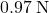. This force would point away from the center of the square (to the left at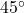above the horizontal direction.)

Explanation:

Coulomb’s constant: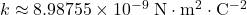.

By Coulomb’s Law, the electrostatic force between two point charges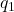and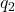that are separated by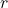(vacuum) would be: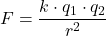.

Consider the charge on the top-left corner of this square.

Apply Coulomb’s Law to find the electrostatic force between this charge and the charge on the lower-left corner.

Convert quantities to standard units: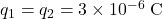.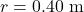.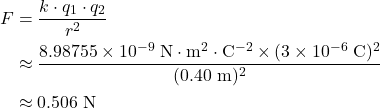.

As the two charges are of the same sign, the electrostatic force on each charge would point away from the other charge. Hence, for the charge on the top-left corner of the square, the electrostatic force from the charge below it would point upwards.

Similarly, the charge to the right of this charge would exert an electrostatic force with the same magnitude (approximately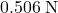) that points leftwards.

For the charge to the lower-right of the top-left charge,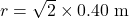. Therefore: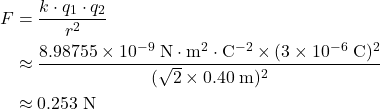.

This force would point to the top-left of the top-left charge, which isabove the horizontal direction. Decompose this force into two components that are normal to one another:

• Horizontal component: approximately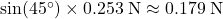.
• Vertical component: approximately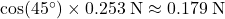Consider the net force on the top-left charge in two components:

• Horizontal component: approximatelyfrom the charge on the top-right corner and approximately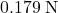from the charge on the lower-right corner. Both components point to the left-hand side.(to the left).
• Vertical component: approximatelyfrom the charge on the lower-left corner and approximatelyfrom the charge on the lower-right corner. Both components point upwards.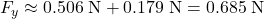(upward).

Combine these two components to find the magnitude of the net force on this charge: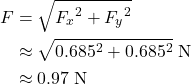.

This force would point to the top-left of this charge (also atabove the horizontal direction, away from the center of the square) because its horizontal and vertical components have the same magnitude.